# How To Simplify Algebraic Expressions Involving Exponents?

Whether you face an algebraic fraction with exponents or without exponents, there is no difference in the solution steps. What we need to mention here is that the types of algebraic fractions in the previous tutorial were all monomials. When the base value has a power of more than one, usually written as a superscript, we approach the simplification process differently.

Algebraic expressions with higher powers are polynomials or exponents. The approach to solving such fractions differ, and in this tutorial, we will look at two ways of simplifying them. The first method would be a simple factorization. The second one using the law of indices is commonly for solving more complex algebraic fractions. We will conclude our discussion by solving some complex fractions with algebraic expressions on the numerator and denominator.

## How to simplify fractions with positive exponents?

Algebraic fractions with exponents are no different from others. The factorization method still applies. For example, if given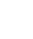as the fraction to simplify, we can proceed as follows: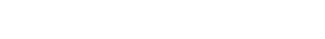After factorization, like terms cancel out. However, this is only applicable if we are dealing with multiplications. It will be wrong to do the same for addition or subtraction.

For example, simplify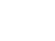This expression is already in its simplest form. We cannot proceed as in the above example. If dealing with additions and subtractions, then pull out the common terms from the numerator and denominator and then cancel out if possible. Let us illustrate that using an example.

Simplify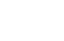### Solution

The term “a” is common, and therefore, we can pull it out as shown: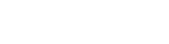when you pull a common term out of the bracket, you reduce the power by one.

Note: Any number whose exponent is zero always equals one. For instance,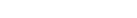Fraction with negative powers

Example: Simplify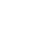Anything at the top with a negative power becomes positive when taken to the denominator and vice versa. So, the above fraction can also take the form of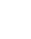. We can easily simplify this fraction by factorization method to get: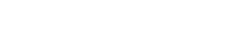### How to simplify fractions using law of indices?

Before looking at an example, let us list down all the laws of indices that we will apply in solving more complex algebraic fractions.We are going to apply the above laws of indices to effortlessly simplify complex algebraic fractions. Let us see a few examples.

Example 1:

Simplify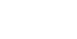### Solution

The above fraction seems too complex to solve by factorization may take you a long time. We are to apply the laws of indices, one by one where applicable, to simplify the problem.

By applying laws number 4 and 5, the fraction becomes: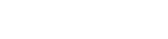We have moved components with negative exponents according to the laws. We are now going to apply law number two to simplify it further.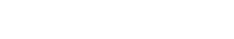The only law of indices that we have not used is number 3. By using it to simplify for the term with “c” as the base, we get: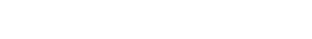We can now factor out 8 to have the fraction in its simplest form.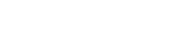Example 2:

Simplify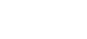### Solution

Let’s recall our laws of indices from number one to 5. Please identify which laws are applicable in each of the following steps:### How to simplify algebraic fractions with polynomial expressions?

If you have an algebraic fraction with polynomial expressions either on the numerator or denominator or both, factor out terms. You may also need to collect like terms. The following identities can help you in working out such problems:By identifying where any of the above identity is applicable in an algebraic expression, you can always factor them and consequently simplify the fraction.

Example 1:

Simplify  latexpage]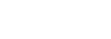### Solution

We can easily see that identity (i) applies to the numerator, and identity (iii) applies to the denominator. Using this knowledge, we can rewrite the fraction and easily simplify it.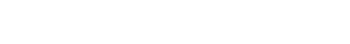Example 2:

Simplify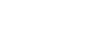### Solution

In this example, we are facing a different type of algebraic expression on the numerator. We cannot apply any of the identities to factor it. The denominator is straightforward by applying identity number (iii) the same we did in example 1.

To factor the numerator, use the distributive property to rewrite 4a. Do that by finding two integers whose sum equals 4, and the product equals 3. The two integers are 3 and 1. Factorization is now easy using that information. factorization is now easier using that information.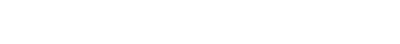Further factorization of the numerator and cancelation of the common terms simplifies the fraction. Note how the numerator turns into a difference of two squares.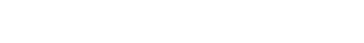### Remarks

You can simplify any algebraic expression no matter how complex it seems, by using the law of indices and the three identities, everything simplifies out effortlessly. Undivided attention is all you need when working with algebra.

If this blog post was helpful, take a look at our Math Tutoring courses which cover these topics and much more.  Our 1-on-1, live and online tutoring will help you master any math subject and ace your upcoming tests.